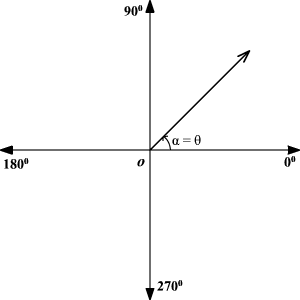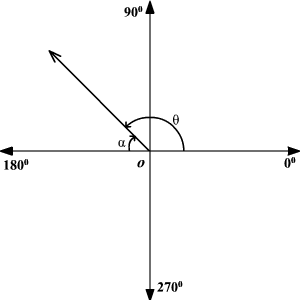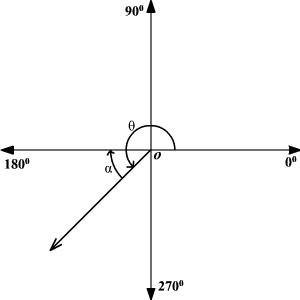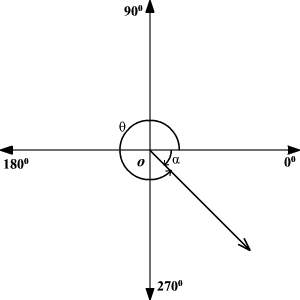# Reference Angle

Let $\theta$  be a non-quadrantal angle in standard position.   Its reference angle is the acute angle $\alpha$ formed by the terminal side of $\theta$ and the horizontal axis.

Quadrant $\text{I}$:

The given angle and the reference angle are the same angle.

$\alpha =\theta$Quadrant $\text{II}$:

$\alpha =\pi -\theta$(radians)

$\alpha =180°-\theta$(degrees)Quadrant $\text{III}$:

$\alpha =\theta -\pi$(radians)

$\alpha =\theta -180°$(degrees)Quadrant $\text{IV}$:

$\alpha =2\pi -\theta$(radians)

$\alpha =360°-\theta$(degrees)